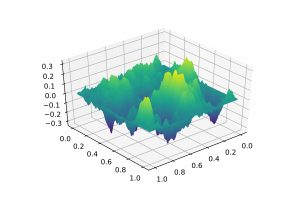# AA1 – Life Sciences

## Stochastic Analysis of Particle Systems: Langevin Dynamics and the Dean-Kawasaki Model

Peter Friz, Ralf Kornhuber, Nicolas Perkowski

Project Members

N.N. (TU), Helena Kremp (FU/HU)

Project Duration

01.08.2019 – 31.07.2022

Located at

FU Berlin

### Description

Naturally emerging models for particle systems in the Life Sciences are often questionable or even meaningless from a mathematical point of view. The goal of this project is to develop mathematically rigorous formulations and solution concepts together with a stochastic and numerical analysis.

### Langevin dynamics for membrane-protein interactions

The interplay of proteins and curvature of biological membranes are well known to regulate cell morphology and a variety of cell functions. Hybrid models describe proteins as rigid particles interacting with a continuous plate-like membrane. They have quite a history in biological physics but have been analyzed only recently from a mathematical point of view.

The analysis is based on a variational formulation in terms of minimization problems for the energy given in terms of the linearized Canham-Helfrich energy in the Monge-Gauge for membrane deformation, and with membrane-particle interactions represented by hard constraints or by penalization.

A crucial aspect of advanced hybrid models concerns ﬂuctuations caused by thermal eﬀects and the surrounding medium. One can derive a formal overdamped Langevin equation to describe the dynamics, but this equation is only well posed in the limit of infinite timescale separation, where the membrane fluctuates much faster than the proteins. Without this assumption, the mathematical meaning of the Langevin equation is dubious due to the inherent irregularity of the problem. The equation even seems far out of reach of novel techniques in the field of stochastic partial differential equations (SPDEs) that are designed specifically to deal with singularities, such as Hairer’s regularity structures.

A natural simplification is to not take the membrane as a minimizer of the energy, but rather to sample it randomly from the Gibbs measure associated to the energy. In the zero temperature limit we would then recover minimizers. Unfortunately, typical realizations of a random membrane sampled from the Gibbs measure are non-differentiable. This leads to the problem of constructing diffusions in non-smooth manifolds, which seems related to the recently constructed Liouville Brownian motion.Typical (non-smooth) sample under the Gibbs measure

### Fluctuating hydrodynamics and Dean-Kawasaki dynamics

Similar mathematical problems arise in fluctuating hydrodynamics, where the aim is to describe the evolution of large (but finite) numbers of interacting particles by means of suitable SPDEs. A typical example is the Dean-Kawasaki equation for the density of particles that appears in fluctuating fluid dynamics. From a mathematical point of view, this equation is severely ill posed due to the irregularity of the noise, unless we carefully interpret it through a martingale problem.

Nonetheless the Dean-Kawasaki equation is useful. For example, it allows us to heuristically guess the large particle number limit, including law of large numbers, central limit theorem, and large deviation rate function. Moreover, the SPDE formulation naturally leads to formal finite element discretizations of the particle dynamics that are successfully applied in practice but without theoretical justification.

Our goal is to rigorously justify the finite element discretization and to derive explicit error bounds. This is a subtle problem, as the martingale problem for the Dean-Kawasaki equation is only well defined after a very fine tuning of the parameters: Recently Konarovskyi, Lehmann and von Renesse showed that slightly changing one of the parameters in the equation leads to an ill posed problem without solutions.

Selected Publications

1. M. Bachmayr, A. Djurdjevac: Multilevel Representations of Isotropic Gaussian Random Fields on the Sphere. arXiv: 2011.06987, 2020.
2. R. Beeson, N. Sri Namachchivaya, N. Perkowski: Approximation of the Filter Equation for Multiple Timescale, Correlated, Nonlinear Systems. arXiv:2010.16401, 2020.
3. C. Bellingeri, A. Djurdjevac, P.K. Friz, and N. Tapia: Transport and continuity equations with (very) rough noise. Partial Differ. Equ. Appl. 2, 49, 2021.
4. L. Church, A. Djurdjevac, C. M. Elliott: A domain mapping approach for elliptic equations posed on random bulk and surface domains, Numerische Mathematik, 146(1), 1-49, 2020.
5. J.D. Deuschel, T. Orenshtein, N. Perkowski: Additive functionals as rough paths. Ann. Probab. 49(3): 1450-1479, 2021.
6. A. Djurdjevac: Linear Parabolic Problems in Random Moving Domains. SIAM/ASA J. Uncertain. Quantification 9 (2021): 848-879
7. P.K. Friz, Y. Kifer: Almost sure diffusion approximation in averaging via rough paths theory. arXiv: 2111.05390, 2021.
8. L. Helfmann, N. Djurdjevac Conrad, A. Djurdjevac, S. Winkelmann, C. Schütte: From interacting agents to density-based modeling with stochastic PDEs. Comm App Math Comp Sci, 1-32, 2021.
9. H. Kremp, N. Perkowski: Multidimensional SDE with distributional drift and Lévy noise. arXiv: 2008.05222, 2020.

Please insert any kind of pictures (photos, diagramms, simulations, graphics) related to the project in the above right field (Image with Text), by choosing the green plus image on top of the text editor. (You will be directed to the media library where you can add new files.)
(We need pictures for a lot of purposes in different contexts, like posters, scientific reports, flyers, website,…
Please upload pictures that might be just nice to look at, illustrate, explain or summarize your work.)